# 2.18 Perform a degrees of freedom analysis for the model inEqs. 2-64 through 2-68. Identify parameters, output variables,and inputs (manipulated and disturbance variables). tions in the inletMixture of A and Bmics of the pro-omatic control is9, CA, TV, p, T2.4 Dynareaction. In other words, the heat of mixing is neg-ligible compared to the heat of reaction.8. Shaft work and heat losses to the ambient can beneglected.decrease inlellllllddisplayed incentration asThe following form of the CSTR energy balanceis convenient for analysis and can be derived fromEqs. 2-62 and 2-63 and Assumptions 1-8 (Fogler, 2006;Russell and Denn, 1972),TR) have wide-embody manySTR models tendtypes of continu-and packed-bedodel provides aing principles forTable 2.3 NcCooling mediumat temperatureT.ParameterVpcdT= wC(T; - T) + (-AHR)Vkc,Figure 2.6 A nonisothermal continuous stirred-tank reactor.dt+ UA(T. – T)(2-68)For these assumptions, the unsteady-state mass balance {for the CSTR iswhere AHR is the heat of reaction per mole of A that isreacted.In summary, the dynamic model of the CSTR consistsof Eqs. 2-62 to 2-64, 2-66, 2-67, and 2-68. This model isnonlinear as a result of the many product terms and Tneexponential temperature dependence of k in Eq. 2-63.Consequently, it must be solved by numerical integra-tion techniques (Fogler, 2006). The CSTR model willbecome considerably more complex if-AHRversible chemicalets to form speciesB. We assume thatd(pV)(2-64)= pq, - pgdtBecause V and p are constant, Eq. 2-64 reduces to450respect to compo-(2-65)q = 9iThus, even though the inlet and outlet flow rates maychange due to upstream or downstream conditions,Eq. 2-65 must be satisfied at all times. In Fig. 2.6, bothflow rates are denoted by the symbol q.For the stated assumptions, the unsteady-state com-ponent balances for species A (in molar concentrationunits) is(2-62)e 400-1. More complicated rate expressions are considered.For example, a mass action kinetics model for asecond-order, irreversible reaction, 2A B, isgiven byper unit volume,units of reciprocaltion of species A.onstant is typicallyature given by the1 = kzc350 ---.(2-69)2. Additional species or chemical reactions areinvolved. If the reaction mechanism involved pro-duction of an intermediate species, 2A B → B,then unsteady-state component balances for bothA and B* would be necessary (to calculate c, andCR), or balances for both A and B could be written(to calculate c, and cB). Information concerningthe reaction mechanisms would also be required.y dea= q(CAi - CA) – VkcA(2-66)300dt(2-63)This balance is a special case of the general componentbalance in Eq. 2-7.Next,balance for the CSTR. But first we make five additionalFigure 2.7 Rea.changes in cooland from 300 toE is the activationThe expressions intheoretical consid-and E are usuallyconsideran unsteady-state energyweReactions involving multiple species are described byhigh-order, highly coupled, nonlinear reaction models,because several component balances must be written.assumptions:1.04. The thermal capacitances of the coolant and thecooling coil wall are negligible compared to thethermal capacitance of the liquid in the tank.5. All of the coolant is at a uniform temperature, T-(That is, the increase in coolant temperature as thecoolant passes through the coil is neglected.)0.9data. Thus, theseobe semi-empiricalin Section 2.2.CSTR is shown in0.8EXAMPLE 2.50.7To illustrate how the CSTR can exhibit nonlinear dynamicbehavior, we simulate the effect of a step change in thecoolant temperature T, in positive and negative directions.Table 2.3 shows the parameters and nominal operatingcondition for the CSTR based on Eqs. 2-66 and 2-68 for theexothermic, irreversible first-order reaction Atwo state variables of the ODES are the concentration ofA (c.) and the reactor temperature T. The manipulatedinput variable is the jacket water temperature, TTwo cases are simulated, one based on increased cool-ing by changing T, from 300 to 290 K and one reducing thecooling rate by increasing T, from 300 to 305 K.These model equations are solved in MATLAB with anumerical integrator (ode15s) over a 10-min horizon. The0.6of pure componentcooling coil is usedt the desired oper-cat that is released0.50.46. The rate of heat transfer from the reactor contentsto the coolant is given by0.30.2-0.1B. Thenitial CSTR model(2-67)Q = UA(T- T)mptions:where U is the overall heat transfer coefficient andA is the heat transfer area. Both of these modelparameters are assumed to be constant.7. The enthalpy change associated with the mixing ofthe feed and the liquid in the tank is negligible com-pared with the enthalpy change for the chemical0.Figure 2.8 React.changes in coolinand product streamsare denoted by p.ctor is kept constantReactant A concentration (mol/L)Reactor temperature (K):

Question
80 views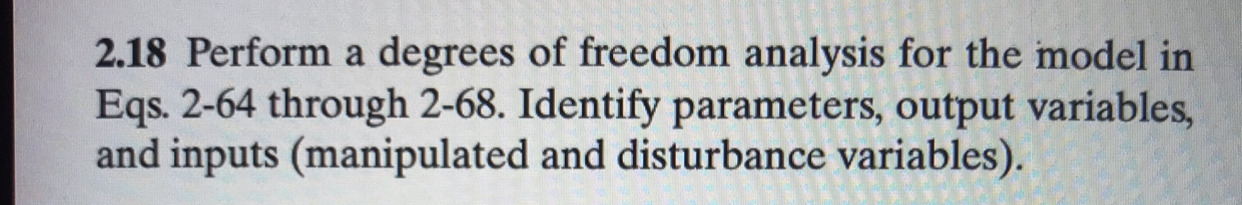help_outlineImage Transcriptionclose2.18 Perform a degrees of freedom analysis for the model in Eqs. 2-64 through 2-68. Identify parameters, output variables, and inputs (manipulated and disturbance variables). fullscreen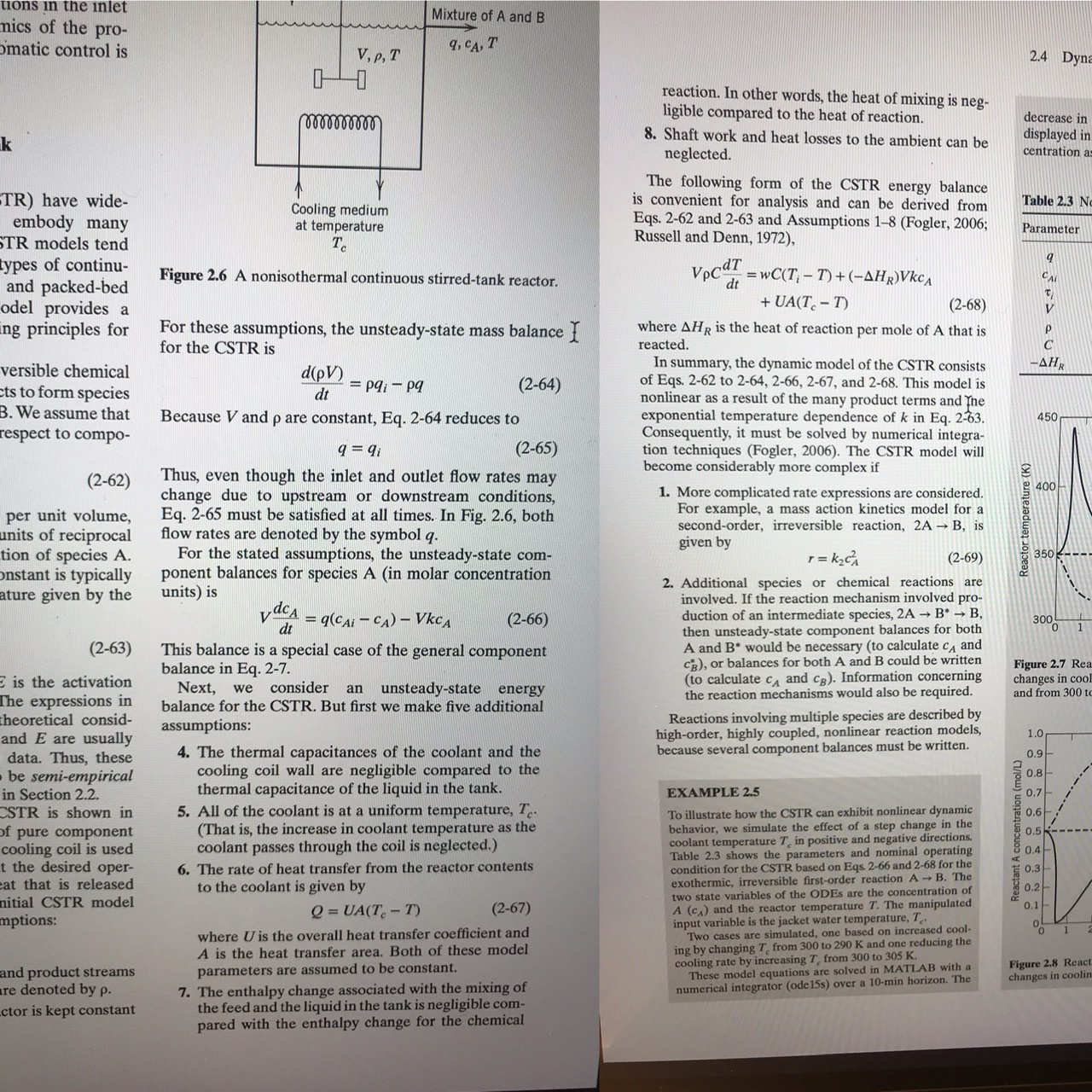help_outlineImage Transcriptionclosetions in the inlet Mixture of A and B mics of the pro- omatic control is 9, CA, T V, p, T 2.4 Dyna reaction. In other words, the heat of mixing is neg- ligible compared to the heat of reaction. 8. Shaft work and heat losses to the ambient can be neglected. decrease in lelllllld displayed in centration as The following form of the CSTR energy balance is convenient for analysis and can be derived from Eqs. 2-62 and 2-63 and Assumptions 1-8 (Fogler, 2006; Russell and Denn, 1972), TR) have wide- embody many STR models tend types of continu- and packed-bed odel provides a ing principles for Table 2.3 Nc Cooling medium at temperature T. Parameter VpcdT = wC(T; - T) + (-AHR)Vkc, Figure 2.6 A nonisothermal continuous stirred-tank reactor. dt + UA(T. – T) (2-68) For these assumptions, the unsteady-state mass balance { for the CSTR is where AHR is the heat of reaction per mole of A that is reacted. In summary, the dynamic model of the CSTR consists of Eqs. 2-62 to 2-64, 2-66, 2-67, and 2-68. This model is nonlinear as a result of the many product terms and Tne exponential temperature dependence of k in Eq. 2-63. Consequently, it must be solved by numerical integra- tion techniques (Fogler, 2006). The CSTR model will become considerably more complex if -AHR versible chemical ets to form species B. We assume that d(pV) (2-64) = pq, - pg dt Because V and p are constant, Eq. 2-64 reduces to 450 respect to compo- (2-65) q = 9i Thus, even though the inlet and outlet flow rates may change due to upstream or downstream conditions, Eq. 2-65 must be satisfied at all times. In Fig. 2.6, both flow rates are denoted by the symbol q. For the stated assumptions, the unsteady-state com- ponent balances for species A (in molar concentration units) is (2-62) e 400- 1. More complicated rate expressions are considered. For example, a mass action kinetics model for a second-order, irreversible reaction, 2A B, is given by per unit volume, units of reciprocal tion of species A. onstant is typically ature given by the 1 = kzc 350 ---. (2-69) 2. Additional species or chemical reactions are involved. If the reaction mechanism involved pro- duction of an intermediate species, 2A B → B, then unsteady-state component balances for both A and B* would be necessary (to calculate c, and CR), or balances for both A and B could be written (to calculate c, and cB). Information concerning the reaction mechanisms would also be required. y dea = q(CAi - CA) – VkcA (2-66) 300 dt (2-63) This balance is a special case of the general component balance in Eq. 2-7. Next, balance for the CSTR. But first we make five additional Figure 2.7 Rea. changes in cool and from 300 to E is the activation The expressions in theoretical consid- and E are usually consider an unsteady-state energy we Reactions involving multiple species are described by high-order, highly coupled, nonlinear reaction models, because several component balances must be written. assumptions: 1.0 4. The thermal capacitances of the coolant and the cooling coil wall are negligible compared to the thermal capacitance of the liquid in the tank. 5. All of the coolant is at a uniform temperature, T- (That is, the increase in coolant temperature as the coolant passes through the coil is neglected.) 0.9 data. Thus, these obe semi-empirical in Section 2.2. CSTR is shown in 0.8 EXAMPLE 2.5 0.7 To illustrate how the CSTR can exhibit nonlinear dynamic behavior, we simulate the effect of a step change in the coolant temperature T, in positive and negative directions. Table 2.3 shows the parameters and nominal operating condition for the CSTR based on Eqs. 2-66 and 2-68 for the exothermic, irreversible first-order reaction A two state variables of the ODES are the concentration of A (c.) and the reactor temperature T. The manipulated input variable is the jacket water temperature, T Two cases are simulated, one based on increased cool- ing by changing T, from 300 to 290 K and one reducing the cooling rate by increasing T, from 300 to 305 K. These model equations are solved in MATLAB with a numerical integrator (ode15s) over a 10-min horizon. The 0.6 of pure component cooling coil is used t the desired oper- cat that is released 0.5 0.4 6. The rate of heat transfer from the reactor contents to the coolant is given by 0.3 0.2- 0.1 B. The nitial CSTR model (2-67) Q = UA(T- T) mptions: where U is the overall heat transfer coefficient and A is the heat transfer area. Both of these model parameters are assumed to be constant. 7. The enthalpy change associated with the mixing of the feed and the liquid in the tank is negligible com- pared with the enthalpy change for the chemical 0. Figure 2.8 React. changes in coolin and product streams are denoted by p. ctor is kept constant Reactant A concentration (mol/L) Reactor temperature (K): fullscreen
check_circle

star
star
star
star
star
1 Rating
Step 1

The degree of freedom analysis of a process model ensures that its model equations are solvable. The expression to calculate the degree of freedom is: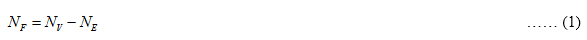Here, NF is the number of degrees of freedom for the process model, N is the total number of process variables, and NE is the total number of independent equations written for the process model.

Step 2

The model given in equations 2-64 through 2-68 is: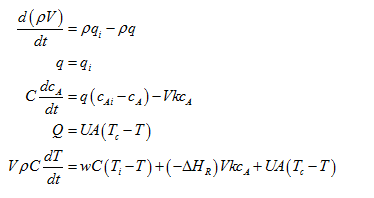Step 3

From the above equations, independent equations that are deduced for the model are: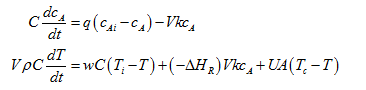...

### Want to see the full answer?

See Solution

#### Want to see this answer and more?

Solutions are written by subject experts who are available 24/7. Questions are typically answered within 1 hour.*

See Solution
*Response times may vary by subject and question.
Tagged in

### Chemical Engineering